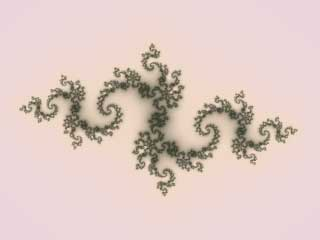# What are fractals?Ultra Fractal creates images of fractals. Fractal images are created by repeatedly calculating a fractal formula. Although these formulas are purely mathematical, the resulting pictures are often very beautiful and complex.

Ultra Fractal goes a long way toward hiding the mathematical stuff. Instead, you focus on the fractals themselves, the way they are combined, and how they are colored. This enables you to turn your fractals into true works of art.

This chapter will explain the basics of fractals, and why they are so interesting.

Next: Self-similarity